# RD Sharma Class 9 Solutions Chapter 17 Constructions Ex 17.2

## RD Sharma Class 9 Solutions Chapter 17 Constructions Ex 17.2

These Solutions are part of RD Sharma Class 9 Solutions. Here we have given RD Sharma Class 9 Solutions Chapter 17 Constructions Ex 17.2

Other Exercises

Question 1.
Find the area of a quadrilateral ABCD in which AB = 3cm, BC = 4cm, CD = 4cm, DA = 5cm and AC = 5cm (NCERT)
Solution:
In the quadrilateral, AC is the diagonal which divides the figure into two triangles
Now in ∆ABC, AB = 3 cm, BC = 4cm, AC = 5cm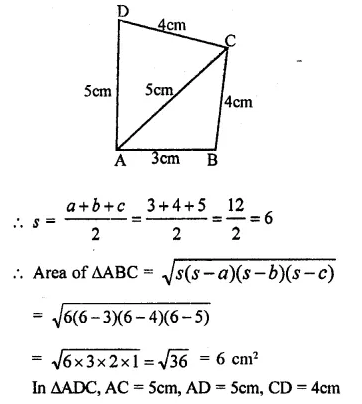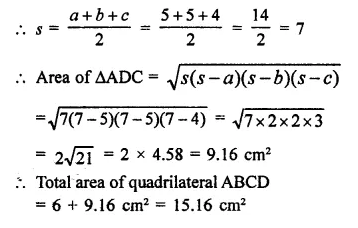Question 2.
The sides of a quadrangular field taken in order are 26 m, 27 m, 7 m and 24 m respectively. The angle contained by the last two sides is a right angle. Find its area.
Solution:
In quad. ABCD, AB = 26 m, BC = 27 m CD = 7m, DA = 24 m, ∠CDA = 90°
Join AC,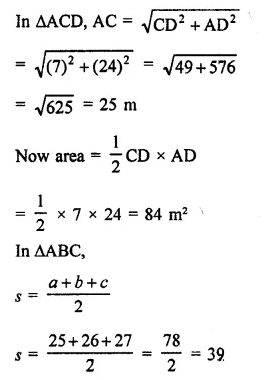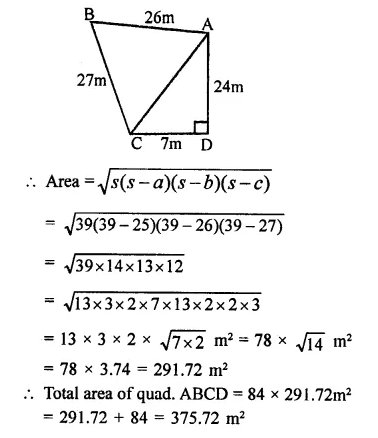Question 3.
The sides of a quadrilateral taken in order are 5, 12, 14 and 15 metres respectively, and the angle contained by the first two sides is a right angle. Find its area.
Solution:
AB = 5m, BC = 12 m, CD = 14m,
DA = 15 m and ∠ABC = 90°
Join AC,
Now in right ∆ABC,
AC² = AB² + BC² = (5)² + (12)²
= 25 + 144 = 169 = (13)²
∴ AC = 13 m
Now area of right ∆ABC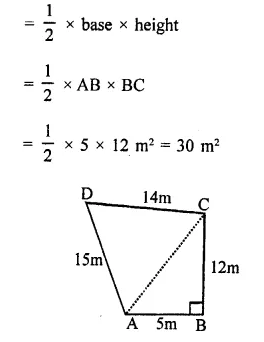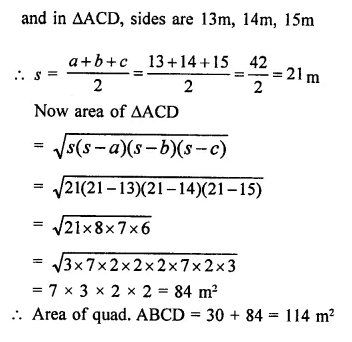Question 4.
A park, in shape of a quadrilateral ABCD, has ∠C = 90°, AB = 9m, BC = 12m, CD = 5m and AD = 8m. How much area does it occupy? (NCERT)
Solution:
AB = 9m, BC = 12m, CD = 5m and
DA = 8m, ∠C = 90°
Join BD,
Now in right ∆BCD,
BD² = BC²+ CD² = (12)² + (5)²
= 144 + 25 = 169 = (13)²
∴ BD = 13m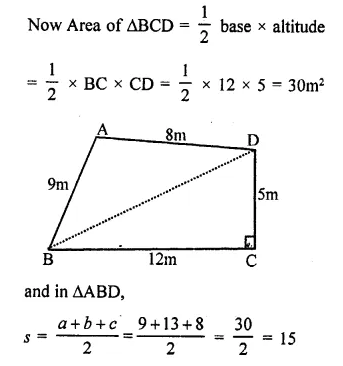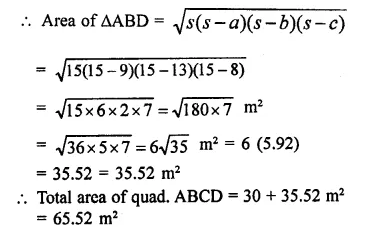Question 5.
Find the area of a rhombus whose perimeter is 80m and one of whose diagonal is 24m.
Solution:
Perimeter of rhombus ABCD = 80 m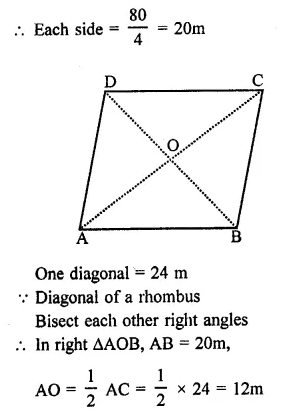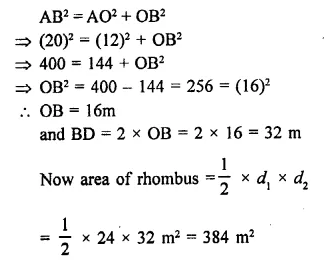Question 6.
A rhombus sheet whose perimeter = 32 m and whose one diagonal is 10 m long, is painted on both sides at the rate of ₹5 per m². Find the cost of painting.
Solution:
Perimeter of the rhombus shaped sheet = 32 m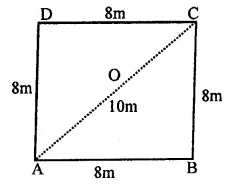∴ Length of each side = $$\frac { 32 }{ 4 }$$ = 8m
and length of one diagonal AC = 10 m
In ∆ABC, sides are 8m, 8m, 10m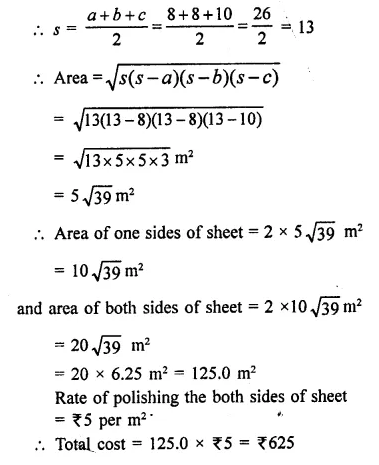Question 7.
Find the area of a quadrilateral ABCD in which AD = 24 cm, ∠BAD = 90° and BCD forms an equilateral triangle whose each side is equal to 26 cm. (Take $$\sqrt { 3 }$$ = 1.73 )
Solution: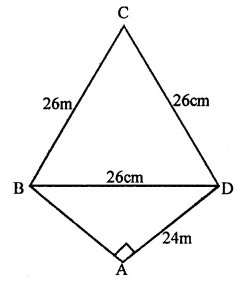BCD is an equilateral triangle with side 26cm
In right ∆ABD,
(26)² = AB² + (24)²
⇒ 676 = AB² + 576
AB² = 676 – 576 = 100 = (10)²
∴ AB = 10cm
Now area of right ∆ABD,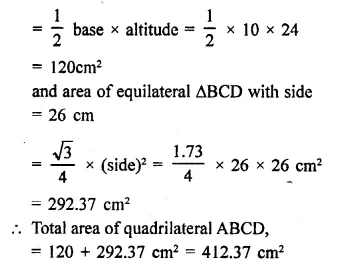Question 8.
Find the area of a quadrilateral ABCD in which AB = 42cm, BC = 21cm, CD = 29 cm, DA = 34 cm and diagonal BD = 20 cm.
Solution:
AB = 42 cm, BC = 21 cm, CD = 29cm DA = 34 cm, BD = 20 cm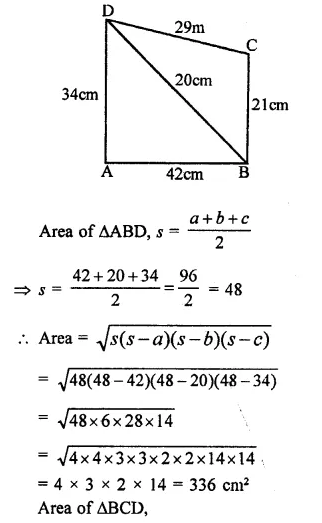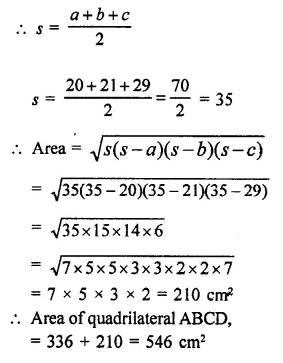Question 9.
The adjacent sides of a parallelogram ABCD measures 34 cm and 20 cm, and the diagonal AC measures 42 cm. Find the area of the parallelogram.
Solution:
In ||gm ABCD,
AB = 34cm, BC = 20 cm
and AC = 42 cm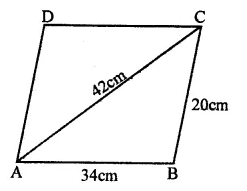∵ The diagonal of a parallelogram divides into two triangles equal in area,
Now area of ∆ABC,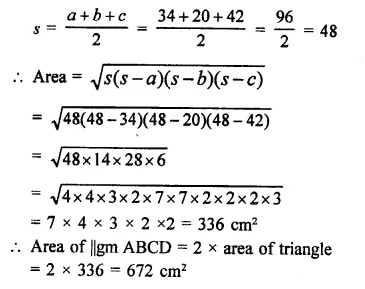Question 10.
Find the area of the blades of the magnetic compass shown in figure. (Take $$\sqrt { 11 }$$ = 3.32).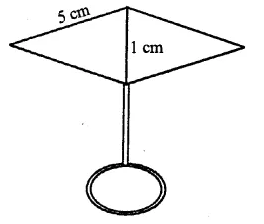Solution:
ABCD is a rhombus with each side 5cm and one diagonal 1cm
Diagonal BD divides into two equal triangles Now area of ∆ABD,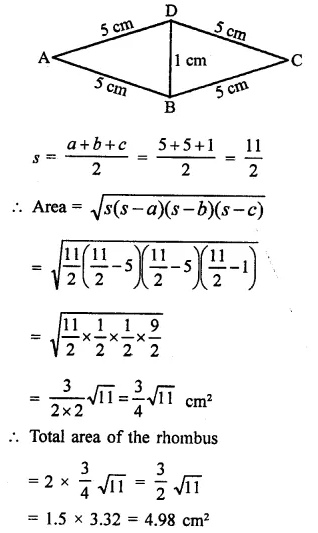Question 11.
A triangle and a parallelogram have the same base and the same area. If the sides of the triangle are 13 cm, 14 cm and 15 cm and the parallelogram stands on the base 14 cm, find the height of the parallelogram.
Solution:
Area of a triangle with same base and area of a 11gm with equal sides of triangle are 13, 14, 15 cm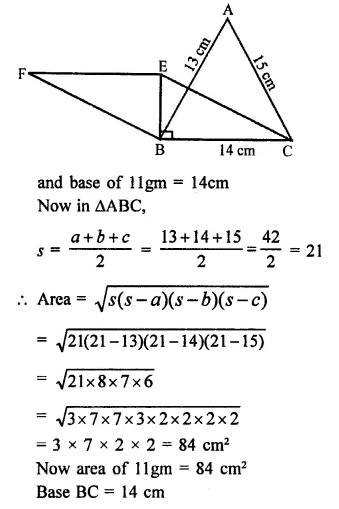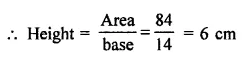Question 12.
Two parallel sides of a trapezium are 60cm and 77 cm and other sides are 25 cm and 26 cm. Find the area of the trapezium.
Solution:
In trapezium ABCD, AB || DC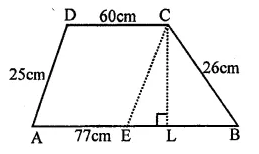AB = 77cm, BC = 26 cm, CD 60cm DA = 25 cm
Through, C, draw CE || DA meeting AB at E
∴ AE = CD = 60 cm and EB = 77 – 60 = 17 cm,
CE = DA = 25 cm
Now area of ∆BCE, with sides 17 cm, 26 cm, 25 cm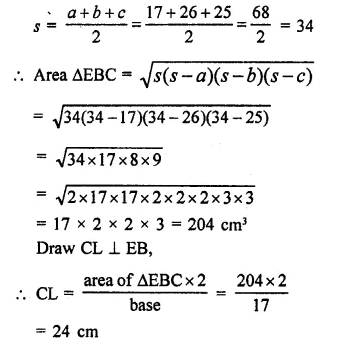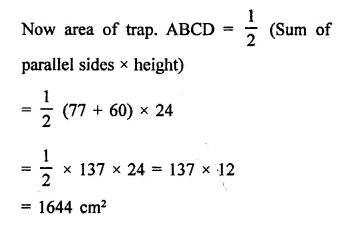Question 13.
Find the perimeter and area of the quadrilateral ABCD in which AB = 17 cm, AD = 9cm, CD = 12cm, ∠ACB = 90° and AC = 15cm.
Solution:
In right ΔABC, ∠ACB = 90°
AB² = AC² + BC²
(17)² = (15)²+ BC² = 289 = 225 + BC²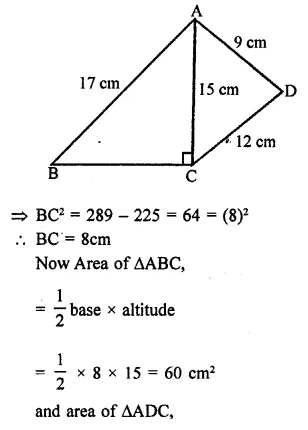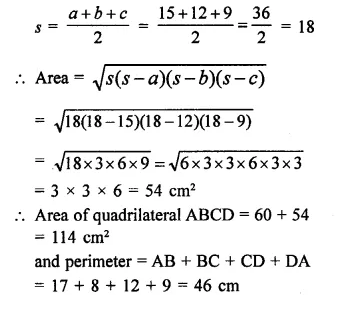Question 14.
A hand fan is made by stitching 10 equal size triangular strips of two different types of paper as shown figure. The dimensions of equal strips are 25 cm, 25 cm and 14 cm. Find the area of each types of paper needed to make the hand fan.
Solution:
In the figure, a hand fan has 5 isosceles and triangle. With sides 25 cm, 25 cm and 14 cm each.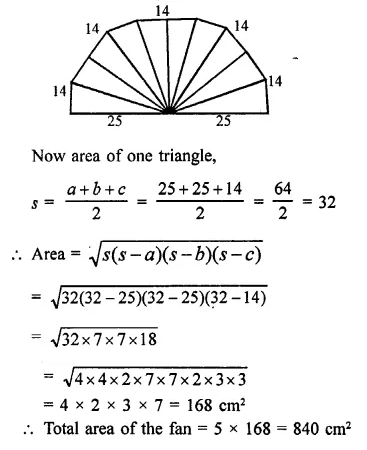Hope given RD Sharma Class 9 Solutions Chapter 17 Constructions Ex 17.2 are helpful to complete your math homework.

If you have any doubts, please comment below. Learn Insta try to provide online math tutoring for you.